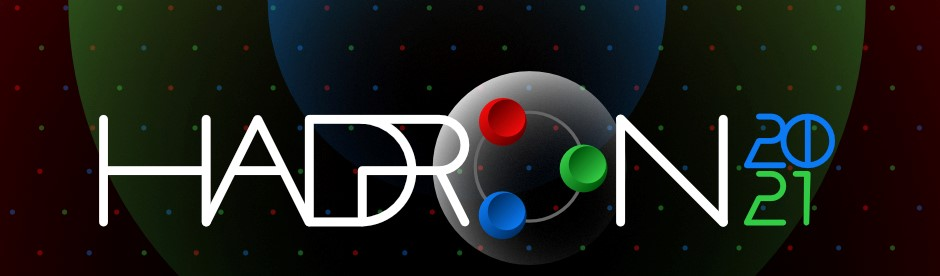19th International Conference on Hadron Spectroscopy and Structure in memoriam Simon Eidelman

26-31 July 2021
Mexico City
Mexico/General timezone
HADRON 2021 is over. Thanks for making it a success!
Home > Timetable > Session details
PDF | iCal

Meson spectroscopy-5

Place

Location: Mexico City
Date: 28 Jul 11:15 - 13:15

Conveners

• Prof. Ryan, Sinead (Trinity College Dublin)

Timetable | Contribution List

Displaying 5 contributions out of 5
Type: Parallel Session: Meson spectroscopy-5
Track: Meson Spectroscopy
We present results to test the limits of the three-particle finite-volume formalisms by extracting hundreds of energies in frames up to, and including, $L^2/(2\pi)^2 \mathbf{P}^2 = 9$ on three CLS ensembles with pion masses of 200, 280, and 340 MeV at fixed lattice spacing. We find the inclusion of d-wave interactions in both the two- and three-particle systems to be necessary to describe our data ... More
Presented by Dr. Andrew HANLON on 28/7/2021 at 12:00
Type: Parallel Session: Meson spectroscopy-5
Track: Meson Spectroscopy
We generalize the Lellouch-Lüscher formalism to decays to three degenerate scalar particles. The formalism allows a lattice calculation of the $K\to3\pi$ weak decay, the isospin-breaking $\eta \to 3 \pi$ QCD transition, and the electromagnetic $\gamma^*\to 3\pi$ amplitudes that enter the calculation of the hadronic vacuum polarization contribution to muonic $g − 2$.
Presented by Prof. Stephen SHARPE on 28/7/2021 at 12:20
Type: Parallel Session: Meson spectroscopy-5
Track: Meson Spectroscopy
We perform Dalitz plot analysis of $\eta_c$ decays to $\eta'K^+K^-$, $\eta'\pi^+\pi^-$, and $\eta\pi^+\pi^-$, based on a data sample of ~470 fb$^{-1}$ collected by the BABAR experiment at the PEP-II collider. We measure the parameters of $K_0^\ast(1430)$ and $K_0^\ast(1950)$ and observe production of $\sigma(600)$, $f_0(1400)$ and $f_0(1710)$ resonances. We observe new resonances decaying to \$\pi ... More
Presented by Dr. Alessandro PILLONI on 28/7/2021 at 12:40
Type: Parallel Session: Meson spectroscopy-5
Track: Meson Spectroscopy
Hadron scattering information can be accessed indirectly from lattice QCD by computing the spectrum of multi-hadron states and connecting to the infinite volume amplitudes via quantization conditions. This methodology was applied successfully for the two-hadron case to extract phase-shifts and resonance parameters. Only recently this methodology was extended to the three-hadron sector: both lattic ... More
Presented by Andrei ALEXANDRU on 28/7/2021 at 11:40
Type: Leading parallel Session: Meson spectroscopy-5
Track: Meson Spectroscopy
I present a lattice-QCD calculation of the max-isospin,3-pion scattering amplitude (3pi+to3pi+).The calculation combines finite-volume energies with a relativistic field-theoretic formalism,required to interpret the results.I will describe the full work-flow required to reach the final amplitude,implemented here for the first time, and discuss the complicated singularities appearing in the latter. ... More
Presented by Maxwell HANSEN on 28/7/2021 at 16:15
Building timetable...